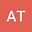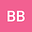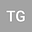Propagation of radius of analyticity for solutions to a fourth order nonlinear Schr\”odinger equation
•••Birilew Belayneh
Bahir Dar University
Author Profile## Abstract

We prove that the uniform radius of spatial analyticity $\sigma(t)$ of solution at time $t$ to the one-dimensional fourth order nonlinear Schr\”odinger equation $$i\partial_tu-\partial_x^4u =|u |^2u$$ cannot decay faster than $1/ \sqrt{t}$ for large $t$, given initial data that is analytic with fixed radius $\sigma_0$. The main ingredients in the proof are a modified Gevrey space, a method of approximate conservation law and a Strichartz estimate for free wave associated with the equation.
26 Jul 2022Submitted to Mathematical Methods in the Applied Sciences
28 Jul 2022Assigned to Editor
28 Jul 2022Submission Checks Completed
02 Sep 2022Reviewer(s) Assigned